# DHARMa - Residual Diagnostics for HierArchical (Multi-level / Mixed) Regression Models

#### 2017-03-10

Abstract

The DHARMa package uses a simulation-based approach to create readily interpretable scaled residuals from fitted generalized linear mixed models. Currently supported are generalized linear mixed models from ‘lme4’ (classes ‘lmerMod’, ‘glmerMod’), generalized additive models (‘gam’ from ‘mgcv’), ‘glm’ (including ‘negbin’ from ‘MASS’, but excluding quasi-distributions) and ‘lm’ model classes. Alternatively, externally created simulations, e.g. posterior predictive simulations from Bayesian software such as ‘JAGS’, ‘STAN’, or ‘BUGS’ can be processed as well. The resulting residuals are standardized to values between 0 and 1 and can be interpreted as intuitively as residuals from a linear regression. The package also provides a number of plot and test functions for typical model misspecification problems, such as over/underdispersion, zero-inflation, and spatial / temporal autocorrelation.

# Motivation

Residual interpretation for generalized linear mixed models (GLMMs) is often problematic. As an example, here two Poisson GLMMs, one that is lacking a quadratic effect, and one that fits the data perfectly. I show three standard residuals diagnostics each. Which is the misspecified model?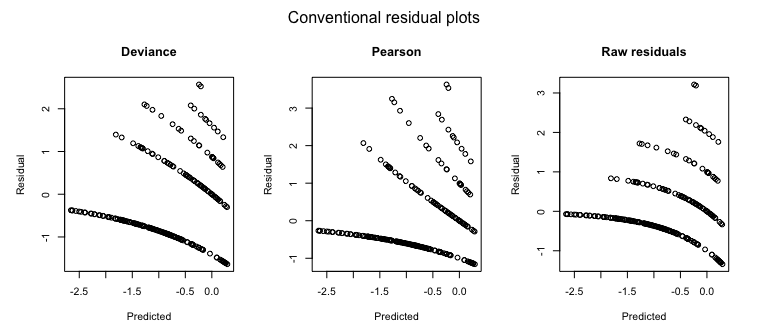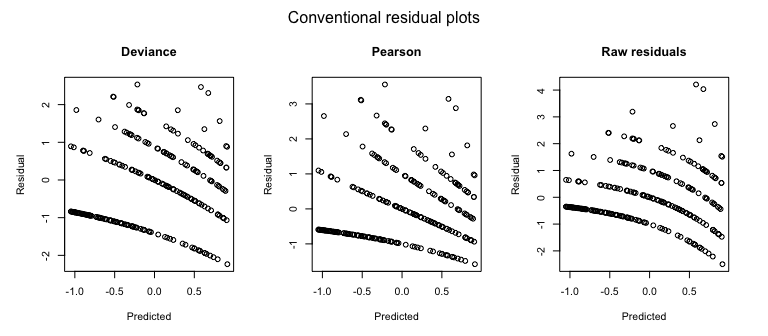Just for completeness - it was the first one. But don’t get too excited if you got it right. Either you were lucky, or you noted that the first model seems a bit overdispersed (range of the Pearson residuals). But even when noting that, would you have added a quadratic effect, instead of adding an overdispersion correction? The point here is that misspecifications in GL(M)Ms cannot reliably be diagnosed with standard residual plots, and GLMMs are thus often not as thoroughly checked as LMs.

One reason why GL(M)Ms residuals are harder to interpret is that the expected distribution of the data changes with the fitted values. Reweighting with the expected variance, as done in Pearson residuals, or using deviance residuals, helps a bit, but does not lead to visually homogenous residuals even if the model is correctly specified. As a result, standard residual plots, when interpreted in the same way as for linear models, seem to show all kind of problems, such as non-normality, heteroscedasticity, even if the model is correctly specified. Questions on the R mailing lists and forums show that practitioners are regularly confused about whether such patterns in GL(M)M residuals are a problem or not.

But even experienced statistical analysts currently have few options to diagnose misspecification problems in GLMMs. In my experience, the current standard practice is to eyeball the residual plots for major misspecifications, potentially have a look at the random effect distribution, and then run a test for overdispersion, which is usually positive, after which the model is modified towards an overdispersed / zero-inflated distribution. This approach, however, has a number of problems, notably:

• Overdispersion often comes from missing or misspecified predictors. Standard residual plots make it difficult to test for residual patterns against the predictors to check for candidates.

• Not all overdispersion is the same. For count data, the negative binomial creates a different distribution than observation-level random effects in the Poisson. Differences between distributional assumptions are not detectable by overdispersion tests, once overdispersion is corrected (because the tests only looks at total dispersion), and nearly impossible to see visually from standard residual plots.

• Dispersion frequently varies with predictors (heteroscedasticity). This can have a significant effect on the inference. While it is standard to tests for heteroscedasticity in linear regressions, heteroscedasticity is currently hardly ever tested for in GLMMs, although it is likely as frequent and influential.

DHARMa aims at solving these problems by creating readily interpretable residuals for generalized linear (mixed) models that are standardized to values between 0 and 1, and that can be interpreted as intuitively as residuals for the linear model. This is achieved by a simulation-based approach, similar to the Bayesian p-value or the parametric bootstrap, that transforms the residuals to a standardized scale. The basic steps are:

1. Simulate new data from the fitted model for the predictor variable combination of each observation.

2. For each observation, calculate the empirical cumulative density function for the simulated data, which describes the expected spread for an observation at the respective point in predictor space, conditional on the fitted model.

3. The residual is defined as the value of the empirical density function at the value of the observed data.

These steps are visualized in the following figure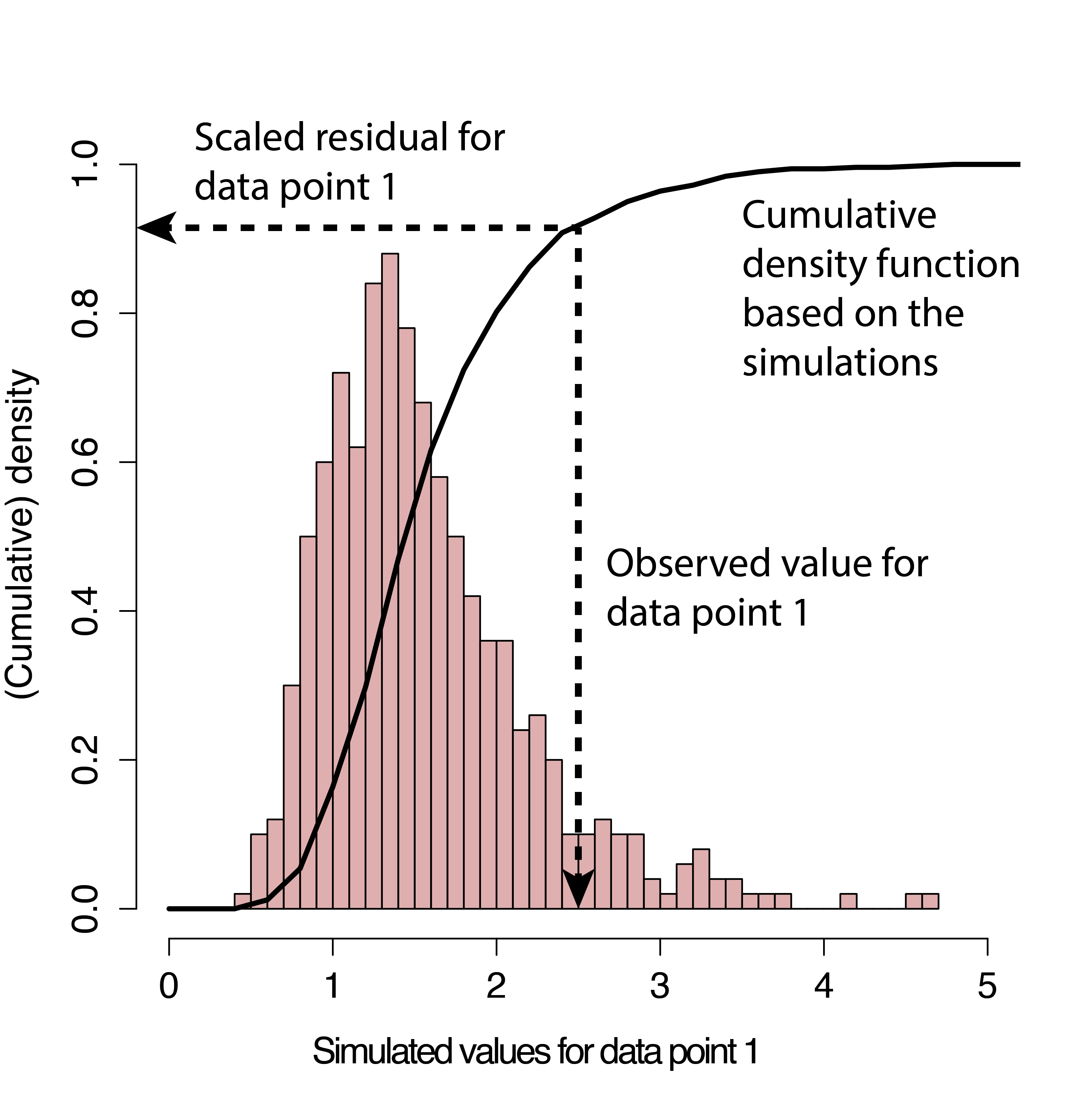The key idea for this definition is that, if the model is correctly specified, then the observed data should look like as if it was created from the fitted model. Hence, for a correctly specified model, all values of the cumulative distribution should appear with equal probability. That means we expect the distribution of the residuals to be flat, regardless of the model structure (Poisson, binomial, random effects and so on).

I currently prepare a more exact statistical justification for the approach in an accompanying paper, but if you must provide a reference in the meantime I would suggest citing

• Dunn, K. P., and Smyth, G. K. (1996). Randomized quantile residuals. Journal of Computational and Graphical Statistics 5, 1-10.

• Gelman, A. & Hill, J. Data analysis using regression and multilevel/hierarchical models Cambridge University Press, 2006

p.s.: DHARMa stands for “Diagnostics for HierArchical Regression Models” – which, strictly speaking, would make DHARM. But in German, Darm means intestines; plus, the meaning of DHARMa in Hinduism makes the current abbreviation so much more suitable for a package that tests whether your model is in harmony with your data:

From Wikipedia, 28/08/16: In Hinduism, dharma signifies behaviours that are considered to be in accord with rta, the order that makes life and universe possible, and includes duties, rights, laws, conduct, virtues and ‘‘right way of living’’.

# Workflow in DHARMa

If you haven’t installed the package yet, either run

``install.packages("DHARMa")``

Or follow the instructions on https://github.com/florianhartig/DHARMa to install a development version.

``````library(DHARMa)
citation("DHARMa")``````
``````##
## To cite package 'DHARMa' in publications use:
##
##   Florian Hartig (2017). DHARMa: Residual Diagnostics for
##   Hierarchical (Multi-Level / Mixed) Regression Models. R package
##   version 0.1.5. http://florianhartig.github.io/DHARMa/
##
## A BibTeX entry for LaTeX users is
##
##   @Manual{,
##     title = {DHARMa: Residual Diagnostics for Hierarchical (Multi-Level / Mixed) Regression Models},
##     author = {Florian Hartig},
##     year = {2017},
##     note = {R package version 0.1.5},
##     url = {http://florianhartig.github.io/DHARMa/},
##   }``````

## Calculating scaled residuals

The scaled (quantile) residuals are calculated with the simulateResiduals() function. The default number of simulations to run is 250, which proved to be a reasonable compromise between computation time and precision, but if high precision is desired, n should be raised to 1000 at least.

``simulationOutput <- simulateResiduals(fittedModel = fittedModel, n = 250)``

What the function does is a) creating n new synthetic datasets by simulating from the fitted model, b) calculates the cumulative distribution of simulated values for each observed value, and c) returning the quantile value that corresponds to the observed value.

For example, a scaled residual value of 0.5 means that half of the simulated data are higher than the observed value, and half of them lower. A value of 0.99 would mean that nearly all simulated data are lower than the observed value. The minimum/maximum values for the residuals are 0 and 1.

The calculated residuals are stored in

``simulationOutput\$scaledResiduals``

As discussed above, for a correctly specified model we would expect

• a uniform (flat) distribution of the overall residuals

• uniformity in y direction if we plot against any predictor.

Note: the expected uniform distribution is the only differences to the linear regression that one has to keep in mind when interpreting DHARMa residuals. If you cannot get used to this and you must have residuals that behave exactly like a linear regression, you can access a normal transformation of the residuals via

``simulationOutput\$scaledResidualsNormal``

These normal residuals will behave exactly like the residuals of a linear regression. However, for reasons of a) numeric stability with low number of simulations and b) my conviction that it is much easier to visually detect deviations from uniformity than normality, I would STRONGLY advice against using this transformation.

## Plotting the scaled residuals

We can get a visual impression of these properties with the plotSimulatedResiduals() function

``plotSimulatedResiduals(simulationOutput = simulationOutput)``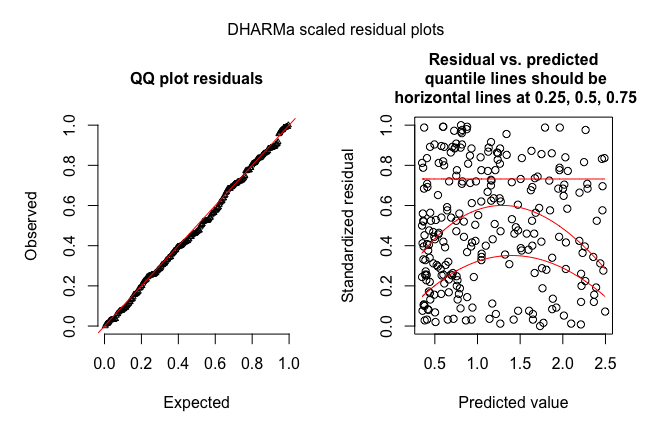which creates a qq-plot to detect overall deviations from the expected distribution, and a plot of the residuals against the predicted value.

To provide a visual aid in detecting deviations from uniformity in y-direction, the plot of the residuals against the predicted values also performs an (optional) quantile regression, which provides 0.25, 0.5 and 0.75 quantile lines across the plots. These lines should be straight, horizontal, and at y-values of 0.25, 0.5 and 0.75. Note, however, that some deviations from this are to be expected by chance, even for a perfect model, especially if the sample size is small.

The quantile regression can be very slow for large datasets. You can chose to use a simpler method with the option quantreg = F.

If you want to plot the residuals against other predictors (highly recommend), you can use the function

``plotResiduals(YOURPREDICTOR, simulationOutput\$scaledResiduals)``

which does the same quantile plot as the main plotting function.

## Formal goodness-of-fit tests on the scaled residuals

To support the visual inspection of the residuals, the DHARMa package provides a number of specialized goodness-of-fit tests on the simulated residuals. For example, the function

``testUniformity(simulationOutput = simulationOutput)``
``````##
##  One-sample Kolmogorov-Smirnov test
##
## data:  simulationOutput\$scaledResiduals
## D = 0.04, p-value = 0.8186
## alternative hypothesis: two-sided``````

runs a KS test to test for overall uniformity of the residuals. There are a number of further tests

• testOverdispersion()
• testZeroinflation()
• testTemporalAutocorrelation()
• testSpatialAutocorrelation()

that basically do what they say. See the help of the functions and further comments below for a more detailed description.

## Simulation options

There are a few important technical details regarding how the simulations are performed, in particular regarding the treatments of random effects and integer responses. I would therefore strongly recommend to read the help of

``?simulateResiduals``

The short summary is this: apart from the number of simulations, there are three important options in the simulateResiduals function

#### Refit

``simulationOutput <- simulateResiduals(fittedModel = fittedModel, refit = T)``
• if refit = F (default), new data is simulated from the fitted model, and residuals are calculated by comparing the observed data to the new data

• if refit = T, a parametric bootstrap is performed, meaning that the model is refit on the new data, and residuals are created by comparing observed residuals against refitted residuals

The second option is much much slower, and therefore not recommended. It is only important for running tests that rely on comparing observed to simulated residuals, e.g. the testOverdispersion function (see below), or if one expects that the tested model is biased and one wants to calculate the expected residuals conditional on this bias (this could make sense in particular for shrinkage estimators that include a purposeful bias, such as random effects or the splines in GAMs). Note also that refit = T can sometimes run into numerical problems, if the fitted model does not converge on the newly simulated data.

#### Random effect simulations

The second option is the treatment of the stochastic hierarchy. In a hierarchical model, several layers of stochasticity are placed on top of each other. Specifically, in a GLMM, we have a lower level stochastic process (random effect), whose result enters into a higher level (e.g. Poisson distribution). For other hierarchical models such as state-space models, similar considerations apply, but the hierarchy can be more complex. When simulating, we have to decide if we want to re-simulate all stochastic levels, or only a subset of those. For example, in a GLMM, it is common to only simulate the last stochastic level (e.g. Poisson) conditional on the fitted random effects, meaning that the random effects are set on the fitted values.

For controlling how many levels should be re-simulated, the simulateResidual function allows to pass on parameters to the simulate function of the fitted model object. Please refer to the help of the different simulate functions (e.g. ?simulate.merMod) for details. For merMod (lme4) model objects, the relevant parameters are “use.u”, and “re.form”, as, e.g., in

``simulationOutput <- simulateResiduals(fittedModel = fittedModel, n = 250, use.u = T)``

If the model is correctly specified and the fitting procedure is unbiased (disclaimer: GLMM estimators are not always unbiased), the simulated residuals should be flat regardless how many hierarchical levels we re-simulate. The most thorough procedure would be therefore to test all possible options. If testing only one option, I would recommend to re-simulate all levels, because this essentially tests the model structure as a whole. This is the default setting in the DHARMa package. A potential drawback is that re-simulating the random effects creates more variability, which may reduce power for detecting problems in the upper-level stochastic processes.

#### Integer treatment / randomization

A third option is the treatment of integer responses. The background of this option is that, for integer-valued variables, some additional steps are neccessary to make sure that the residual distribution becomes flat (essentially, we have to smoothen away the integer nature of the data). The idea is explained in

• Dunn, K. P., and Smyth, G. K. (1996). Randomized quantile residuals. Journal of Computational and Graphical Statistics 5, 1-10.

The simulateResiduals function will automatically check if the family is integer valued, and apply randomization if that is the case. I see no reason why one would not want to randomize for an integer-valued function, so the parameter should usually not be changed.

## Using external simulations (e.g. from Bayesian software)

As mentioned earlier, the quantile residuals defined in DHARMa are the frequentist equivalent of the so-called “Bayesian p-values”, i.e. residuals created from posterior predictive simulations in a Bayesian analysis.

To make the plots and tests in DHARMa also available for Bayesian analysis, DHARMa provides the option to convert externally created posterior predictive simulations into a DHARMa object

``res = createDHARMa(scaledResiduals = posteriorPredictiveSimulations, simulatedResponse = medianPosteriorPredictions, observedResponse = observations, integerResponse = ?)``

What is provided as simulatedResponse is up to the user, but median posterior predictions seem most sensible to me. Note: as DHARMa doesn’t know the fitted model, it is important in this function to specify the integerResponse option by hand (see simulateResiduals for details). After the conversion, all DHARMa plots can be used, however, note that Bayesian p-values != DHARMA residuals, because in the Bayesian analysis, parameters are varied as well.

## Reproducibility notes

If you running a stochastic algorithms such as the code in DHARMa, you should always set or record your random seed to make your final results reproducible (otherwise, results will change slightly every time you run the code). Do this via

``set.seed(123)``

However, I do not recommend to do this from the start - it’s useful for you to see that results in DHARMa may change slightly if you re-run the script. Just for the final result, the seed should be set or recorded to ensure complete reproducibility. In addition to that (general advice)

``sessionInfo()``

will lists the version number of R and all loaded packages - it is always wise to record this if producing results with R.

# Visual diagnostics and tests of common misspecification problems

In all plots / tests that were shown so far, the model was correctly specified, resulting in “perfect” residual plots. In this section, we discuss how to recognize and interpret model misspecifications in the scaled residuals.

## Overdispersion / underdispersion

The most common concern for GLMMs is overdispersion, underdispersion and zero-inflation.

Over/underdispersion refers to the phenomenon that residual variance is larger/smaller than expected under the fitted model. Over/underdispersion can appear for any distributional family with fixed variance, in particular for Poisson and binomial models.

A few general rules of thumb

• You can detect overdispersion / zero-inflation only AFTER fitting the model
• Overdispersion is more common than underdispersion
• If overdispersion is present, confidence intervals tend to be too narrow, and p-values to small. The opposite is true for underdispersion
• A common reason for overdispersion is a misspecified model. When overdispersion is detected, one should therefore first search for problems in the model specification (e.g. by plotting residuals against predictors with DHARMa), and only if this doesn’t lead to success, overdispersion corrections such as individual-level random effects or changes in the distribution should be applied

#### An example of overdispersion

This this is how overdispersion looks like in the DHARMa residuals

``````testData = createData(sampleSize = 500, overdispersion = 2, family = poisson())
fittedModel <- glmer(observedResponse ~ Environment1 + (1|group) , family = "poisson", data = testData)

simulationOutput <- simulateResiduals(fittedModel = fittedModel)
plotSimulatedResiduals(simulationOutput = simulationOutput)``````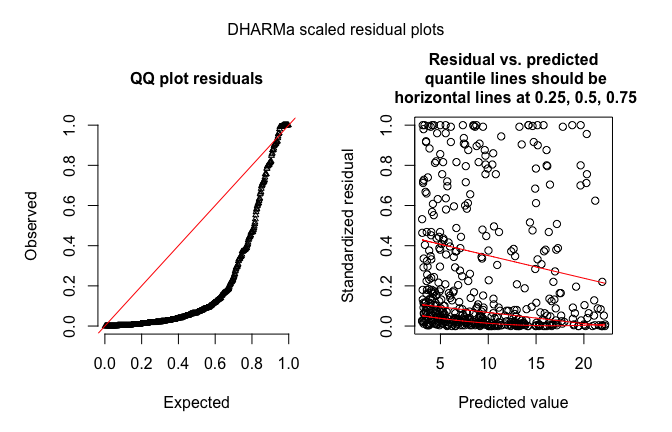Note that we get more residuals around 0 and 1, which means that more residuals are in the tail of distribution than would be expected under the fitted model.

#### An example of underdispersion

This is an example of underdispersion

``````testData = createData(sampleSize = 500, intercept=0, fixedEffects = 2, overdispersion = 0, family = poisson(), roundPoissonVariance = 0.001, randomEffectVariance = 0)
fittedModel <- glmer(observedResponse ~ Environment1 + (1|group) , family = "poisson", data = testData)

summary(fittedModel)``````
``````## Generalized linear mixed model fit by maximum likelihood (Laplace
##   Approximation) [glmerMod]
##  Family: poisson  ( log )
## Formula: observedResponse ~ Environment1 + (1 | group)
##    Data: testData
##
##      AIC      BIC   logLik deviance df.resid
##    971.9    984.5   -482.9    965.9      497
##
## Scaled residuals:
##     Min      1Q  Median      3Q     Max
## -0.5990 -0.3553 -0.1159  0.2015  1.0618
##
## Random effects:
##  Groups Name        Variance  Std.Dev.
##  group  (Intercept) 4.205e-18 2.05e-09
## Number of obs: 500, groups:  group, 10
##
## Fixed effects:
##              Estimate Std. Error z value Pr(>|z|)
## (Intercept)  -0.22089    0.06276   -3.52 0.000432 ***
## Environment1  2.31910    0.09002   25.76  < 2e-16 ***
## ---
## Signif. codes:  0 '***' 0.001 '**' 0.01 '*' 0.05 '.' 0.1 ' ' 1
##
## Correlation of Fixed Effects:
##             (Intr)
## Environmnt1 -0.839``````
``````# plotConventionalResiduals(fittedModel)

simulationOutput <- simulateResiduals(fittedModel = fittedModel)
plotSimulatedResiduals(simulationOutput = simulationOutput)``````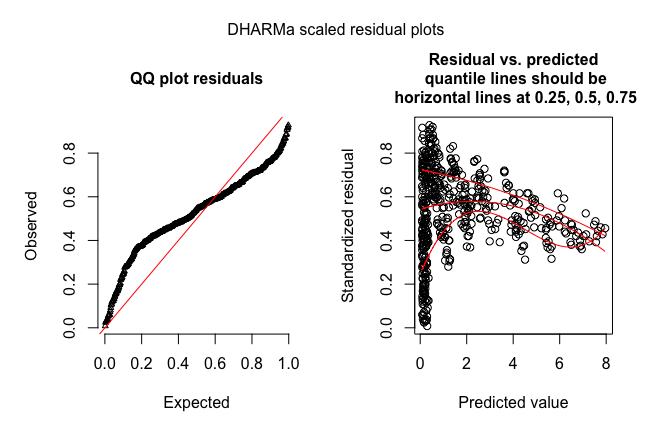``testUniformity(simulationOutput = simulationOutput)``
``````##
##  One-sample Kolmogorov-Smirnov test
##
## data:  simulationOutput\$scaledResiduals
## D = 0.194, p-value < 2.2e-16
## alternative hypothesis: two-sided``````

Here, we get too many residuals around 0.5, which means that we are not getting as many residuals as we would expect in the tail of the distribution than expected from the fitted model.

#### Testing for over/underdispersion

Although, as discussed above, over/underdispersion will show up in the residuals, and it’s possible to detect it with the testUniformity function, simulations show that this test is less powerful than more targeted tests.

DHARMa therefore contains several specific overdispersion test that compares the dispersion of simulated residuals to the observed residuals. Those are

1. A parametric overdispersion test
2. A non-parametric test on the simulated residuals
3. A non-parametric overdispersion test on the re-fitted residuals.

Technically, you can call these tests as follows:

``````# Option 1
testOverdispersionParametric(fittedModel)``````
``````##
##  Chisq test for overdispersion in GLMMs
##
## data:  poisson
## dispersion = 0.15809, pearSS = 78.57000, rdf = 497.00000, p-value
## = 1
## alternative hypothesis: true dispersion greater 1``````
``````# Option 2
testOverdispersion(simulationOutput)``````
``````##
##  DHARMa nonparametric overdispersion test via IQR of scaled
##  residuals against IQR expected under uniform
##
## data:  simulationOutput
## dispersion = 0.50833, p-value = 1
## alternative hypothesis: overdispersion``````
``````# Option 3
simulationOutput2 <- simulateResiduals(fittedModel = fittedModel, refit = T, n = 20)
testOverdispersion(simulationOutput2)``````
``````##
##  DHARMa nonparametric overdispersion test via comparison to
##  simulation under H0 = fitted model
##
## data:  simulationOutput2
## dispersion = 0.15978, p-value = 1
## alternative hypothesis: overdispersion``````

Power simulations (figure below, see extended simulations here) show that option 2 is generally not preferable. I only kept it in the package for testing and future improvements.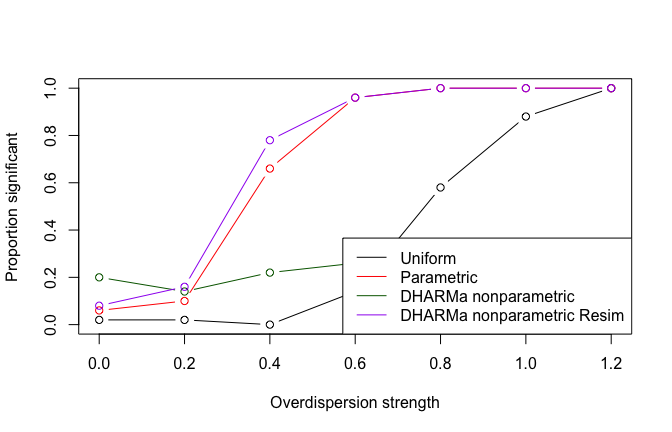Comparison of power from simulation studies

Option 1 and 3 are substantially more powerful than the standard uniform test. The parametric alternative (option 1) has the advantage that it is fast, while option 3 might have slightly higher power and all other advantages of a non-parametric test, i.e. it should be still reliable for situations where distributional assumptions are violated. For normal use, I recommend using the parametric test.

A word of warning that applies also to all other tests that follow: significance in hypothesis tests depends on at least 2 ingredients: strenght of the signal, and number of data points. Hence, the p-value alone is not a good indicator of the extent to which your residuals deviate from assumptions. Specifically, if you have a lot of data points, residual diagnostics will nearly inevitably become significant, because having a perfectly fitting model is very unlikely. That, however, doesn’t neccessarily mean that you need to change your model. The p-values confirm that there is a deviation from your null hypothesis. It is, however, in your discretion to decide whether this deviation is worth worrying about. If you see a dispersion parameter of 1.01, I would not worry, even if the test is significant. A significant value of 5, however, is clearly a reason to move to a model that accounts for overdispersion.

## Zero-inflation

A common special case of overdispersion is zero-inflation, which is the situation when more zeros appear in the observation than expected under the fitted model. Zero-inflation requires special correction steps.

#### An example of zero-inflation

Here an example of a typical zero-inflated count dataset, plotted against the environmental predictor

``````testData = createData(sampleSize = 500, intercept = 2, fixedEffects = c(1), overdispersion = 0, family = poisson(), quadraticFixedEffects = c(-3), randomEffectVariance = 0, pZeroInflation = 0.6)

par(mfrow = c(1,2))
plot(testData\$Environment1, testData\$observedResponse, xlab = "Envrionmental Predictor", ylab = "Response")
hist(testData\$observedResponse, xlab = "Response", main = "")``````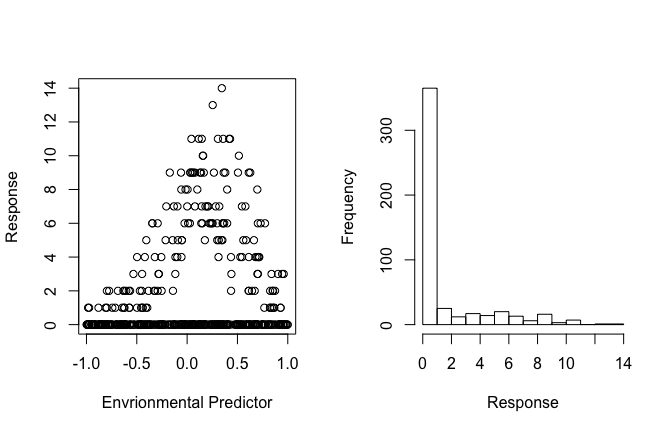We see a hump-shaped dependence of the environment, but with too many zeros.

#### Zero-inflation in the scaled residuals

In the normal residual, plots, zero-inflation will look pretty much like overdispersion

``````fittedModel <- glmer(observedResponse ~ Environment1 + I(Environment1^2) + (1|group) , family = "poisson", data = testData)

simulationOutput <- simulateResiduals(fittedModel = fittedModel)
plotSimulatedResiduals(simulationOutput = simulationOutput)``````